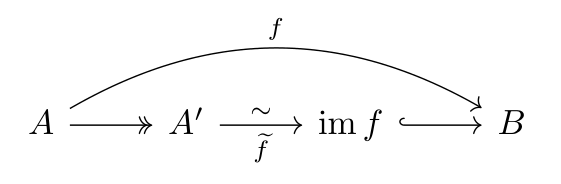Show Question
Math and science::Algebra::Aluffi

# Canonical decomposition

Any function $$f : A \to B$$ can be decomposed into a surjection, followed by an isomorphism, followed by an injection.

The surjection will be a projection to a partition of $$A$$ induced by $$f$$. The isomorphism will be from this set to the image $$\operatorname{im} f$$. The injection will be the inclusion back to $$B$$.

#### Equivalence relation induced by a function

Let $$f : A \to B$$ be a function. $$f$$ induces an equivalence relation $$\sim$$ on $$A$$ as follows: For all $$a', a'' \in A$$,

$a' \sim a'' \iff f(a') = f(a'').$

### Theorem. Canonical decomposition

Let $$f : A \to B$$ be any function, and define $$\sim$$ as above. Then $$f$$ decomposes as follows:First we have the canonical projection $$A \to A/\sim$$. The last function is the inclusion $$\operatorname{im} f \subseteq B$$. The bijection in the middle is defined as:

$\widetilde{f}([a]_{\sim}) = f(a)$

This theorem states that the above diagram for $$f$$'s decomposition computes and that $$\widetilde{f}$$ is a valid function and is a bijection.

#### Well-defined

There is ambiguity as to which elements of $$A$$ are chosen to represent the equivalence classes in $$A \ \sim$$. As a consequence, this theorem should be accompanied by a proof to show that any choice available leads to the same result. Such a proof is said to be a verification of the theorem being well-defined. Aluffi does exactly this, and it is a good example of such proofs.

Aluffi
p15